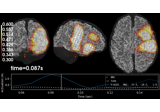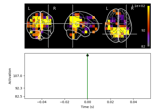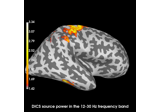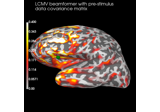# mne.beamformer.make_lcmv¶

mne.beamformer.make_lcmv(info, forward, data_cov, reg=0.05, noise_cov=None, label=None, pick_ori=None, rank='info', weight_norm='unit-noise-gain-invariant', reduce_rank=False, depth=None, inversion='matrix', verbose=None)[source]

Compute LCMV spatial filter.

Parameters
infoinstance of `Info`

The measurement info to specify the channels to include. Bad channels in info[‘bads’] are not used.

forwardinstance of `Forward`

Forward operator.

data_covinstance of `Covariance`

The data covariance.

reg`float`

The regularization for the whitened data covariance.

noise_covinstance of `Covariance`

The noise covariance. If provided, whitening will be done. Providing a noise covariance is mandatory if you mix sensor types, e.g. gradiometers with magnetometers or EEG with MEG.

labelinstance of `Label`

Restricts the LCMV solution to a given label.

pick_ori

For forward solutions with fixed orientation, None (default) must be used and a scalar beamformer is computed. For free-orientation forward solutions, a vector beamformer is computed and:

• `None`

Orientations are pooled after computing a vector beamformer (Default).

• `'normal'`

Filters are computed for the orientation tangential to the cortical surface.

• `'max-power'`

Filters are computed for the orientation that maximizes power.

• `'vector'`

Keeps the currents for each direction separate

rank`None` | ‘info’ | ‘full’ | `dict`

This controls the rank computation that can be read from the measurement info or estimated from the data. When a noise covariance is used for whitening, this should reflect the rank of that covariance, otherwise amplification of noise components can occur in whitening (e.g., often during source localization).

`None`

The rank will be estimated from the data after proper scaling of different channel types.

`'info'`

The rank is inferred from `info`. If data have been processed with Maxwell filtering, the Maxwell filtering header is used. Otherwise, the channel counts themselves are used. In both cases, the number of projectors is subtracted from the (effective) number of channels in the data. For example, if Maxwell filtering reduces the rank to 68, with two projectors the returned value will be 66.

`'full'`

The rank is assumed to be full, i.e. equal to the number of good channels. If a `Covariance` is passed, this can make sense if it has been (possibly improperly) regularized without taking into account the true data rank.

`dict`

Calculate the rank only for a subset of channel types, and explicitly specify the rank for the remaining channel types. This can be extremely useful if you already know the rank of (part of) your data, for instance in case you have calculated it earlier.

This parameter must be a dictionary whose keys correspond to channel types in the data (e.g. `'meg'`, `'mag'`, `'grad'`, `'eeg'`), and whose values are integers representing the respective ranks. For example, `{'mag': 90, 'eeg': 45}` will assume a rank of `90` and `45` for magnetometer data and EEG data, respectively.

The ranks for all channel types present in the data, but not specified in the dictionary will be estimated empirically. That is, if you passed a dataset containing magnetometer, gradiometer, and EEG data together with the dictionary from the previous example, only the gradiometer rank would be determined, while the specified magnetometer and EEG ranks would be taken for granted.

The default is `'info'`.

weight_norm

Can be:

• `None`

The unit-gain LCMV beamformer 1 will be computed.

• `'unit-noise-gain'`

The unit-noise gain minimum variance beamformer will be computed (Borgiotti-Kaplan beamformer) 1, which is not rotation invariant when `pick_ori='vector'`. This should be combined with `stc.project('pca')` to follow the definition in 1.

• `'nai'`

The Neural Activity Index 2 will be computed, which simply scales all values from `'unit-noise-gain'` by a fixed value.

• `'unit-noise-gain-invariante'`

Compute a rotation-invariant normalization using the matrix square root. This differs from `'unit-noise-gain'` only when `pick_ori='vector'`, creating a solution that:

1. Is rotation invariant (`'unit-noise-gain'` is not);

2. Satisfies the first requirement from 1 that `w @ w.conj().T == I`, whereas `'unit-noise-gain'` has non-zero off-diagonals; but

3. Does not satisfy the second requirement that `w @ G.T = θI`, which arguably does not make sense for a rotation-invariant solution.

Defaults to `'unit-noise-gain-invariant'`.

reduce_rankbool

If True, the rank of the denominator of the beamformer formula (i.e., during pseudo-inversion) will be reduced by one for each spatial location. Setting `reduce_rank=True` is typically necessary if you use a single sphere model with MEG data.

Changed in version 0.20: Support for reducing rank in all modes (previously only supported `pick='max_power'` with weight normalization).

depth

How to weight (or normalize) the forward using a depth prior. If float (default 0.8), it acts as the depth weighting exponent (`exp`) to use None is equivalent to 0, meaning no depth weighting is performed. It can also be a `dict` containing keyword arguments to pass to `mne.forward.compute_depth_prior()` (see docstring for details and defaults). This is effectively ignored when `method='eLORETA'`.

Changed in version 0.20: Depth bias ignored for `method='eLORETA'`.

New in version 0.18.

inversion‘single’ | ‘matrix’

This determines how the beamformer deals with source spaces in “free” orientation. Such source spaces define three orthogonal dipoles at each source point. When `inversion='single'`, each dipole is considered as an individual source and the corresponding spatial filter is computed for each dipole separately. When `inversion='matrix'`, all three dipoles at a source vertex are considered as a group and the spatial filters are computed jointly using a matrix inversion. While `inversion='single'` is more stable, `inversion='matrix'` is more precise. See section 5 of 3. Defaults to `'matrix'`.

New in version 0.21.

verbose

If not None, override default verbose level (see `mne.verbose()` and Logging documentation for more). If used, it should be passed as a keyword-argument only.

Returns
filtersinstance of `Beamformer`

Dictionary containing filter weights from LCMV beamformer. Contains the following keys:

‘kind’str

The type of beamformer, in this case ‘LCMV’.

‘weights’array

The filter weights of the beamformer.

‘data_cov’instance of Covariance

The data covariance matrix used to compute the beamformer.

‘noise_cov’instance of Covariance | None

The noise covariance matrix used to compute the beamformer.

‘whitener’None | ndarray, shape (n_channels, n_channels)

Whitening matrix, provided if whitening was applied to the covariance matrix and leadfield during computation of the beamformer weights.

‘weight_norm’str | None

Type of weight normalization used to compute the filter weights.

‘pick-ori’None | ‘max-power’ | ‘normal’ | ‘vector’

The orientation in which the beamformer filters were computed.

‘ch_names’list of str

Channels used to compute the beamformer.

‘proj’array

Projections used to compute the beamformer.

‘is_ssp’bool

If True, projections were applied prior to filter computation.

‘vertices’list

Vertices for which the filter weights were computed.

‘is_free_ori’bool

If True, the filter was computed with free source orientation.

‘n_sources’int

Number of source location for which the filter weight were computed.

‘src_type’str

Type of source space.

‘source_nn’ndarray, shape (n_sources, 3)

For each source location, the surface normal.

‘proj’ndarray, shape (n_channels, n_channels)

Projections used to compute the beamformer.

‘subject’str

The subject ID.

‘rank’int

The rank of the data covariance matrix used to compute the beamformer weights.

‘max-power-ori’ndarray, shape (n_sources, 3) | None

When pick_ori=’max-power’, this fields contains the estimated direction of maximum power at each source location.

‘inversion’‘single’ | ‘matrix’

Whether the spatial filters were computed for each dipole separately or jointly for all dipoles at each vertex using a matrix inversion.

Notes

The original reference is 2.

To obtain the Sekihara unit-noise-gain vector beamformer, you should use `weight_norm='unit-noise-gain', pick_ori='vector'` followed by `vec_stc.project('pca', src)`.

Changed in version 0.21: The computations were extensively reworked, and the default for `weight_norm` was set to `'unit-noise-gain-invariant'`.

References

1(1,2,3,4)

Kensuke Sekihara and Srikantan S. Nagarajan. Adaptive Spatial Filters for Electromagnetic Brain Imaging. Series in Biomedical Engineering. Springer, Berlin; Heidelberg, 2008. ISBN 978-3-540-79369-4 978-3-540-79370-0. doi:10.1007/978-3-540-79370-0.

2(1,2)

Barry D. Van Veen, Wim van Drongelen, Moshe Yuchtman, and Akifumi Suzuki. Localization of brain electrical activity via linearly constrained minimum variance spatial filtering. IEEE Transactions on Biomedical Engineering, 44(9):867–880, 1997. doi:10.1109/10.623056.

3

Marijn van Vliet, Mia Liljeström, Susanna Aro, Riitta Salmelin, and Jan Kujala. Analysis of functional connectivity and oscillatory power using DICS: from raw MEG data to group-level statistics in Python. bioRxiv, 2018. doi:10.1101/245530.

## Examples using `mne.beamformer.make_lcmv`¶